# NATA Aesthetic Sensitivity Test Sample Paper 2

## 40 Questions MCQ Test NATA - Sample Paper & Mock Tests | NATA Aesthetic Sensitivity Test Sample Paper 2

Description
Attempt NATA Aesthetic Sensitivity Test Sample Paper 2 | 40 questions in 80 minutes | Mock test for JEE preparation | Free important questions MCQ to study NATA - Sample Paper & Mock Tests for JEE Exam | Download free PDF with solutions
QUESTION: 1

Solution:
QUESTION: 2

Solution:
QUESTION: 3

### Identify the given image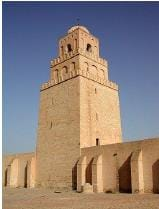Solution:

The Great Mosque of Kairouan, also known as the Mosque of Uqba, is a mosque situated in the UNESCO World Heritage town of Kairouan, Tunisia and is one of the most impressive and largest Islamic monuments in North Africa.

QUESTION: 4

"Form follows function" said by

Solution:
QUESTION: 5

The depression in the brick is called as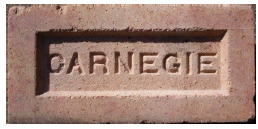Solution:
QUESTION: 6

The dome of rashtrapati bhawan is influenced by

Solution:
QUESTION: 7

Identify the given material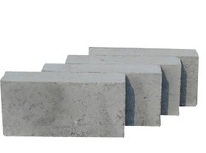Solution:
QUESTION: 8

The colour of pure cement is

Solution:
QUESTION: 9

Pennzoil place was designed by?

Solution:
QUESTION: 10

Count the no of triangles in the given image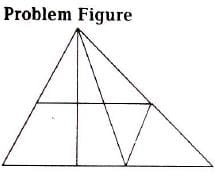Solution:
QUESTION: 11

Identify the series which follows next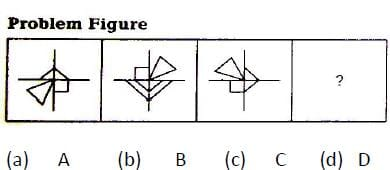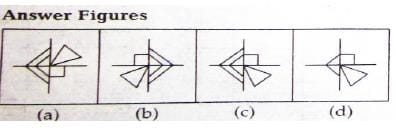Solution:
QUESTION: 12

A particle has two velocity v1 andv2 .its resultant velocity is equal to v1 in magnitude .the angle which the new resultant makes with v2 when v1 is doubled is

Solution:
QUESTION: 13

The law presumes that anyone entering a contract has _____.

Solution:
QUESTION: 14

Identify the give image .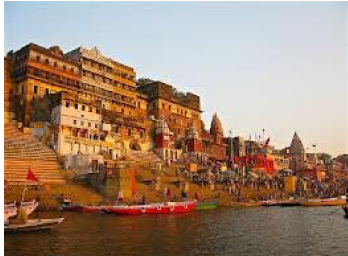Solution:
QUESTION: 15

how many pillars are there in Parliament house

Solution:
QUESTION: 16

Identify the type of staircase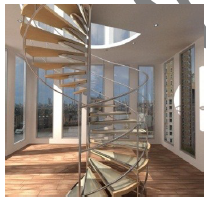Solution:
QUESTION: 17

Give the full form of P.C.C

Solution:
QUESTION: 18

To which style does Taj Mahal belongs to

Solution:
QUESTION: 19

Identify the given type of brick bonding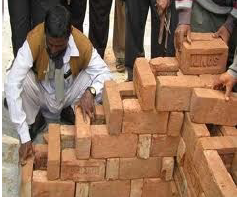Solution:
QUESTION: 20

What follows next ?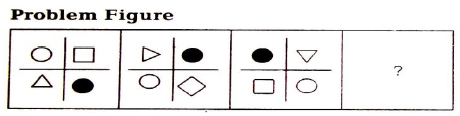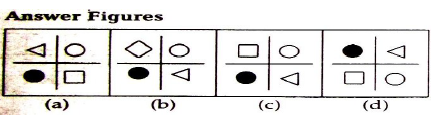Solution:
QUESTION: 21

Which of the following country has the maximum number of tall buildings ?

Solution: China has the largest number of tall buildings in the world, surpassing that of the United States (700+) and Japan (230+) combined.As of early 2017, China has more than 1400 skyscrapers above 150 meters (492 ft) of which 48 are super-tall 300 meters (984 ft and above).
QUESTION: 22

Which one of the answer figure shows the correct view of the 3-d problem figure after the problem figure opened up .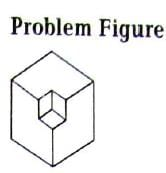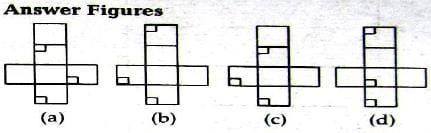Solution:

QUESTION: 23

Which city is known as pink city

Solution:
QUESTION: 24

Meenaskhiamman temple consist of how many pillars

Solution:
QUESTION: 25

Ribbon building bleongs to which style

Solution:
QUESTION: 26

The value of sin6` sin42` sin66` sin78` is

Solution:
LHS
= (cos 6) (cos 42)(cos 66) (cos 78)
= (1/4) * [2cos6 cos66] * [2cos42 cos78]
= (1/4) * (cos72 + cos60) * (cos120 + cos36)
= (1/4) (cos72 + 1/2) * (- 1/2 + cos36)
= (1/4) [- 1/4 + (1/2) (cos36 - cos72) + cos36cos72]
= (1/4) [- 1/4 + sin54sin18 + sin54 sin18]
= (1/4) [- 1/4 + 2sin54 sin18 cos18 / cos18]
= (1/4) [- 1/4 + 2sin54 sin36 / cos18]
= (1/4) [- 1/4 + (cos18 - cos90) / 2cos18]
= (1/4) [- 1/4 + 1/2]
= 1/16
= RHS
QUESTION: 27

Which is known as the temple town of tamilnadu

Solution:
QUESTION: 28

Who is not an architect among the following ?

Solution:
QUESTION: 29

How many petals are there in lotus temple ?

Solution:

D option should be changed to 27.

There are 27 marble petals in the lotus temple.

QUESTION: 30

The grand indian Golden temple located at

Solution:
QUESTION: 31

Acoustics denotes

Solution:
QUESTION: 32

The green building rating system of india is known as

Solution:

GRIHA is correct. GRIHA stands for Green Rating for Integrated Habitat Assessment.

LEED is an internationally recognized green building certification system.

Hence A is the correct answer.

QUESTION: 33

Which of the following architect does many fluidic building types ?

Solution:
QUESTION: 34

Count the number of surface in view given below.